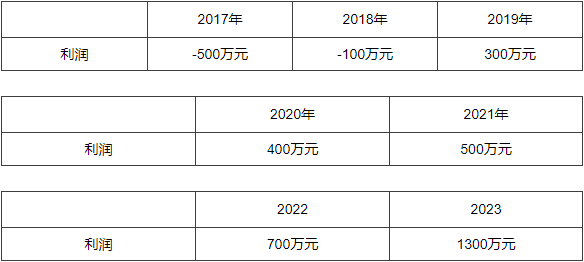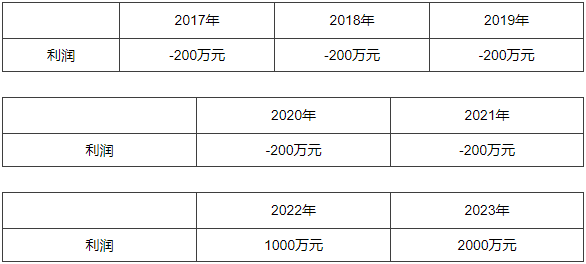## 10分钟了解筹建期纳税筹划！2008年实施的企业所得税法未对筹建期的开办费具体规定。但在《国家税务总局关于企业所得税若干税务事项衔接问题的通知》（国税函〔200998号）对筹建期进行补充。

【模式1：企业逐年盈利且能保持】

 年份 2017 2018 2019 利润 1000万元 1200万元 1400万元

2017年应纳税所得额=1000-（筹建期900+1500=-1400（万元）

2018年应纳税所得额=1200-（以前年度可弥补亏损额1400=-200（万元）

2019年应纳税所得额=1400-（以前年度可弥补亏损额200=1200（万元）

2017年应纳税所得额=1000-（摊销额800=200（万元）

2018年应纳税所得额=1200-（摊销额800=400（万元）

2019年应纳税所得额=1400-（摊销额800=600（万元）

【模式2：企业先亏损后盈利】2017年税收利润=-500-（筹建期900+1500=-2900（万元）

2018年税收利润=-100-（以前年度可弥补亏损额2900=-3000（万元）

2019年税收利润=300-（以前年度可弥补亏损额3000=-2700（万元）

2020年税收利润=400-（以前年度可弥补亏损额2700=-2300（万元）

2021年税收利润=500-（以前年度可弥补亏损额2300=-1800（万元）

2022年税收利润=700-（以前年度可弥补亏损额1800=-1100（万元）

2023年应纳税所得额=1300-（以前年度可弥补亏损额100★=1200（万元）

（解释：因为2023年的盈利仅能弥补至2018年度的亏损，所以2017年的亏损2900万元只弥补了2019~2022年的盈利共计1900万元，浪费了1000万元的亏损额度。）

2017年税收利润=-500-（筹建期摊销额800=-1300（万元）

2018年税收利润=-100-（以前年度可弥补亏损额1300+摊销额800=-2200（万元）

2019年税收利润=300-（以前年度可弥补亏损额2200+摊销额800=-2700（万元）

2020年税收利润=400-（以前年度可弥补亏损额2700=-2300（万元）

2021年税收利润=500-（以前年度可弥补亏损额2300=-1800（万元）

2022年税收利润=700-（以前年度可弥补亏损额1800=-1100（万元）

2023年应纳税所得额=1300-（以前年度可弥补亏损额1100△=200（万元）

（解释：2017年的亏损1300万元通过20192022年的盈利弥补完毕，因为筹建期开办费长期待摊三年的原因，分解了另外两笔800万元的摊销额至2018年与2019年，从而使2023年依然可以弥补筹建期年度的开办费支出）

【模式3：企业正式经营起五年亏损后盈利】2017年税收利润=-200-（筹建期900+1500=-2600（万元）

2018年税收利润=-200-（以前年度可弥补亏损额2600=-2800（万元）

2019年税收利润=-200-（以前年度可弥补亏损额2800=-3000（万元）

2020年税收利润=-200-（以前年度可弥补亏损额3000=-3200（万元）

2021年税收利润=-200-（以前年度可弥补亏损额3200=-3400（万元）

2022年税收利润=1000-（以前年度可弥补亏损额3400=-2400（万元）

2023年应纳税所得额=2000-（以前年度可弥补亏损额800★=1200（万元）

（解释：因为2023年的盈利仅能弥补前五个年度亏损，共计800万元，所以2017年的亏损2600万元只弥补了2022年的盈利1000万元，浪费了1600万元的亏损额度。）

2017年税收利润=-200-（筹建期摊销额800=-1000（万元）

2018年税收利润=-200-（以前年度可弥补亏损额1000+摊销额800=-1200（万元）

2019年税收利润=-200-（以前年度可弥补亏损额1200+摊销额800=-2200（万元）

2020年税收利润=-200-（以前年度可弥补亏损额2200=-2400（万元）

2021年税收利润=-200-（以前年度可弥补亏损额2400=-2600（万元）

2022年税收利润=1000-（以前年度可弥补亏损额2600=-1600（万元）

2023年应纳税所得额=2000-（以前年度可弥补亏损额1600△=400（万元）

（解释：2017年的亏损1000万元通过2022年的盈利弥补完毕，因为筹建期开办费长期待摊三年的原因，分解了另外两笔800万元的摊销额至2018年与2019年，从而使2023年依然可以弥补1819年分摊的筹建期年度的开办费支出共计1600万元）